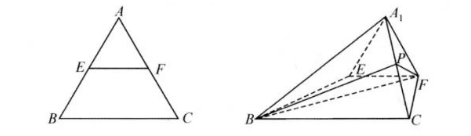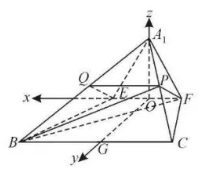(1) 证明: $F P / /$ 平面 $A_1 B E$;
(2) 若平面 $A_1 E F \perp$ 平面 $E F C B$, 求直线 $A_1 F$ 与平面 $B F P$ 所成的角的正弦值.(1) 连接 $A_1 B$ 的中点 $Q$ ，则有 $P Q / / B C$ ，且 $P Q=\frac{1}{2} B C$ ，同理 $E F / / B C$ ， 且 $E F=\frac{1}{2} B C$ ，故 $P Q / / E F$ ，且 $P Q=E F$ ，

(2) 以 $E F$ 中点 $O$ 为原点， $E F$ 为 $y$ 轴， $E F$ 和 $B C$ 的垂直平分线为 $x$ 轴， $A O$ 为 $z$ 轴，建立 空间直角坐标系，则可得 $A_1(0,0, \sqrt{3}), F(0,1,0), B(\sqrt{3},-2,0), C(\sqrt{3}, 2,0), F(0,1,0)$ ， 则由 $P$ 为 $A_1 C$ 中点，故 $P\left(\frac{\sqrt{3}}{2}, 1, \frac{\sqrt{3}}{2}\right)$

，则 $x: y: z=\sqrt{3}: 1: \sqrt{3}$ ，故取 $\vec{n}=(\sqrt{3}, 1, \sqrt{3})$ ，